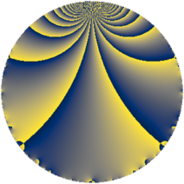# Properties

 Label 154.2.cLevel $154$ Weight $2$ Character orbit 154.c Rep. character $\chi_{154}(153,\cdot)$ Character field $\Q$ Dimension $8$ Newform subspaces $1$ Sturm bound $48$ Trace bound $0$

# Related objects

## Defining parameters

 Level: $$N$$ $$=$$ $$154 = 2 \cdot 7 \cdot 11$$ Weight: $$k$$ $$=$$ $$2$$ Character orbit: $$[\chi]$$ $$=$$ 154.c (of order $$2$$ and degree $$1$$) Character conductor: $$\operatorname{cond}(\chi)$$ $$=$$ $$77$$ Character field: $$\Q$$ Newform subspaces: $$1$$ Sturm bound: $$48$$ Trace bound: $$0$$

## Dimensions

The following table gives the dimensions of various subspaces of $$M_{2}(154, [\chi])$$.

Total New Old
Modular forms 28 8 20
Cusp forms 20 8 12
Eisenstein series 8 0 8

## Trace form

 $$8 q - 8 q^{4} - 16 q^{9} + O(q^{10})$$ $$8 q - 8 q^{4} - 16 q^{9} + 4 q^{11} + 8 q^{14} - 16 q^{15} + 8 q^{16} - 4 q^{22} + 32 q^{23} + 16 q^{36} - 8 q^{37} - 24 q^{42} - 4 q^{44} + 40 q^{49} - 56 q^{53} - 8 q^{56} - 24 q^{58} + 16 q^{60} - 8 q^{64} - 24 q^{67} + 24 q^{70} + 16 q^{71} + 4 q^{77} + 40 q^{78} + 80 q^{81} + 16 q^{86} + 4 q^{88} - 24 q^{91} - 32 q^{92} + 8 q^{93} - 92 q^{99} + O(q^{100})$$

## Decomposition of $$S_{2}^{\mathrm{new}}(154, [\chi])$$ into newform subspaces

Label Dim $A$ Field CM Traces $q$-expansion
$a_{2}$ $a_{3}$ $a_{5}$ $a_{7}$
154.2.c.a $8$ $1.230$ 8.0.$$\cdots$$.1 None $$0$$ $$0$$ $$0$$ $$0$$ $$q+\beta _{1}q^{2}-\beta _{2}q^{3}-q^{4}+\beta _{6}q^{5}-\beta _{3}q^{6}+\cdots$$

## Decomposition of $$S_{2}^{\mathrm{old}}(154, [\chi])$$ into lower level spaces

$$S_{2}^{\mathrm{old}}(154, [\chi]) \cong$$ $$S_{2}^{\mathrm{new}}(77, [\chi])$$$$^{\oplus 2}$$